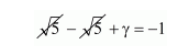# If two zeros x3 + x2 − 5x − 5 are 5–√ and −5–√`
Question:

If two zeros $x^{3}+x^{2}-5 x-5$ are $\sqrt{5}$ and $-\sqrt{5}$, then its third zero is

(a) 1

(b) $-1$

(c) 2

(d) $-2$

Solution:

Let $\alpha=\sqrt{5}$ and $\beta=-\sqrt{5}$ be the given zeros and $\gamma$ be the third zero of $x^{3}+x^{2}-5 x-5=0$ then

By using $\alpha+\beta+\gamma=\frac{-\text { Coefficient of } x^{2}}{\text { Coefficient of } x^{3}}$

$\alpha+\beta+\gamma=\frac{+(+1)}{1}$

$\alpha+\beta+\gamma=-1$

By substituting $\alpha=\sqrt{5}$ and $\beta=-\sqrt{5}$ in $\alpha+\beta+\gamma=-1$$\gamma=-1$

Hence, the correct choice is (b)